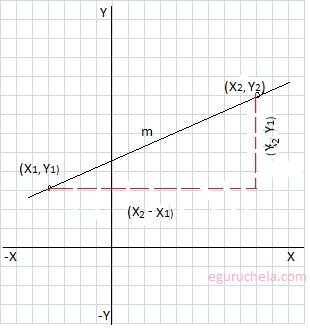### Two Point form Calculator

Enter the values for x and y coordinates for two points in a straight line and calculator find the equation of the straight line passing through the two given points.X1: Y1: X2: Y2: Equation :

To Calculate Equation of a straight line passing
through two points using follwoing formula:
$$\frac{(y-y_1)}{(y_2-y_1)} = \frac{(x-x_1)}{(x_2-x_1)}$$ Let's take the example of line passing through two point (-4, 3) and (9, 8):
Now apply the given value of (x1, y1) and (x2, y2) in the formula:
$$\frac{(y-y_1)}{(y_2-y_1)} = \frac{(x-x_1)}{(x_2-x_1)}$$ $$\frac{(y-3)}{(8-3)} = \frac{(x-(-4))}{(9-(-4))}$$ $$\frac{(y-3)}{(5)} = \frac{(x+4)}{(9+4))}$$ $$\frac{(y-3)}{5} = \frac{(x+4)}{13}$$ $$(y-3) \times{13} =(x+4)\times {5}$$ $$13y-39 =5x+20$$ $$13y-5x= 20 + 39$$ $$13y-5x= 59$$ $$-5x + 13y = 59$$